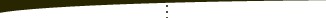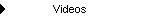About   Products   Support   Videos   Purchase   ContactThe Breakout Bulletin The following article was originally published in the November 2010 issue of The Breakout Bulletin.   Detecting Nonrandomness in the Markets Economists have long debated whether stock market prices change randomly or are at least partially predictable. The random walk hypothesis says that stock market prices change in a random fashion, making prediction impossible. However, more recent research has uncovered a variety of inefficiencies in the markets that disprove the random walk hypothesis. Certainly, any trader who has followed the markets for any length of time, let alone successfully traded them, doesn't need an academic study to tell them the markets are not entirely random. Nonetheless, there's some benefit to being able to demonstrate it mathematically.   The autocorrelation is a statistical measure that is often used to detect nonrandomness in noisy data. For example, if a sine wave is hidden in a noisy electromagnetic signal, the autocorrelation might be used to detect it. The autocorrelation measures the degree of correspondence between a signal and an offset version of the signal. Technically, the autocorrelation is the correlation coefficient of a signal and the signal delayed by a time increment called the lag. The correlation coefficient, and therefore the autocorrelation, returns a value in the range -1 to +1. Values close to either -1 or +1 indicate a high degree of nonrandomness. Values close to zero indicate randomness.   When applying the autocorrelation to market prices, we want to analyze the price changes, rather than the price itself. Price changes often appear to be random. If the price changes have any serial correlation, it will show up in the autocorrelation. In this case, the lag is the number of bars the series of price changes is offset when comparing it to the original series.   For example, consider the following series of price changes:   0, -4, 0.5, 0.5, 3.25, 9.75, 4.5, 21.25, 3.5, -2, -9, 3, -3, -15.5, 0.25, -21, 2.75, 20.25, 0.5   These were obtained by subtracting the prior close from the current close for a short segment of daily bars in the E-mini S&P 500 futures. The autocorrelation at lag 1 for these data would be the correlation coefficient of the following two series:   -4, 0.5, 0.5, 3.25, 9.75, 4.5, 21.25, 3.5, -2, -9, 3, -3, -15.5, 0.25, -21, 2.75, 20.25, 0.5   and   0, -4, 0.5, 0.5, 3.25, 9.75, 4.5, 21.25, 3.5, -2, -9, 3, -3, -15.5, 0.25, -21, 2.75, 20.25   Notice that the second series is just the first one offset by one bar.   The correlation coefficient is defined as the covariance divided by the variance. TradeStation's EasyLanguage contains functions for calculating both the covariance and variance of arrays, so these can be used to calculate the autocorrelation. For example, Value1 = CovarArray(DeltaP, DeltaPLag, NBars); Value2 = VarianceArray(DeltaP, NBars, 1); AutoCorr = 0; If Value2 > 0 then    AutoCorr = Value1/Value2; Above, DeltaP is the array of price changes over the past NBars bars, and DeltaPLag is the array of price changes starting Lag bars back. The complete indicator code is available online on the download page.   In addition to calculating the autocorrelation value itself, it's also necessary to determine the level at which the value is statistically significant. There are several references available online that provide the appropriate formula, such as http://en.wikipedia.org/wiki/Correlogram and http://www.ltrr.arizona.edu/~dmeko/notes_3.pdf. An approximate formula for the 95% confidence bands is: ± 2/sqrt(NBars) where "sqrt" is the square root operator. For example, for NBars equal to 100, the confidence bands are +0.2 and -0.2. That means any value of autocorrelation greater than or equal to 0.2 or less than or equal to -0.2 is considered statistically significant.   I created two versions of the autocorrelation indicator for TradeStation. Both versions plot a line for the autocorrelation value and lines for the confidence limits. Any value outside the confidence limits is colored differently to highlight the fact that it's statistically significant. The first indicator, simply called "Autocorrelation" calculates the autocorrelation of closing price changes over the past NBars bars at lag Lag, where NBars and Lag are inputs. For example, you could use it to plot the autocorrelation over the past 30 bars with a lag of 2.   The other version, named AutocorrMax, is designed to detect significant autocorrelation values for a range of lags from 1 to the input value MaxLag. For example, if MaxLag is 20, the indicator will calculate the autocorrelation for lags 1, 2, 3, ..., 20. The largest autocorrelation (absolute) value is recorded and plotted.   Nonrandomness in the E-mini S&P To illustrate the use of the autocorrelation indicators, I applied them to daily bars of the E-mini S&P 500 futures from 5/17/2006 to 11/19/2010. The application of AutocorrMax is more interesting in that it finds areas where the autocorrelation is significant for any of the lag values from 1 to MaxLag. As a result, there are more statistically significant values than from looking only at a specific lag. I applied this indicator with a MaxLag value of 20 and NBars equal to 100. This means it calculated the autocorrelations over the prior 100 bars for lags from 1 to 20.Figure 1. Maximum autocorrelation values over the past 100 bars for lags 1 to 20. Green indicates statistical significance.   A sample of the E-mini S&P chart is shown in Fig 1. When the maximum autocorrelation value over the different lags is statistically significant (i.e., above the upper band or below the lower band), the indicator plots the value in green. Where the green line crosses from one band to the other, as shown above, the maximum autocorrelation changes from positive to negative from one bar to the next.   Significantly positive values of autocorrelation indicate that the price changes are positively correlated. In other words, a positive price change tends to lead to another positive price change over the lag period. Significantly negative autocorrelations tended to be more prevalent in the chart. A typical period of statistically significant negative correlation is shown below in Fig. 2.Figure 2. Maximum autocorrelation values over the past 100 bars for lags 1 to 20. Green indicates statistical significance.   As shown above, the maximum autocorrelation stays significantly negative for several months. Negative autocorrelation means that the price changes tend to reverse. For example, a positive price change tends to precede a negative price change, and vice-versa.   To estimate how much of the E-mini S&P's price behavior is nonrandom, I saved the indicator values to a file. I opened the file in Excel and tabulated all values that were statistically significant. I found that 62% of price bars in the file had a significant level of autocorrelation, either positive or negative. By this measure, then, 38% of the price changes are random and 62% are nonrandom. It's likely, however, that repeating the calculation with different look-back lengths (NBars) would yield different results.   Keep in mind that the AutocorrMax indicator, as shown in the figures, doesn't show us which lag was statistically significant. It may be that the autocorrelation was significant for several lags or just one. If you want to know which lag was responsible for the significance, you can plot the Autocorrelation indicator for individual lags to test which ones are significant.   Although I didn't test this, the autocorrelation indicators could potentially be used as trading filters. For example, you might try restricting trend trades to periods where the maximum autocorrelation over a range of lags, as in the AutocorrMax indicator, is significantly positive. Likewise, a counter-trend system might benefit by filtering entries to periods where the autocorrelation is negative.   Conclusions The autocorrelation calculation lends support to one of the foundations of technical trading; namely, that the markets contain nonrandom price behavior that can be exploited. Calculating the autocorrelation for a range of lags allows us to uncover a wider range of nonrandomness than focusing on a single lag.   Applying the autocorrelation indicator to the E-mini S&P revealed more periods of negative correlation than positive correlation. It also suggested that there are extended periods of significant autocorrelation and that, overall, the E-mini S&P has a substantial amount of nonrandomness.     Mike Bryant Breakout Futures     HYPOTHETICAL OR SIMULATED PERFORMANCE RESULTS HAVE CERTAIN INHERENT LIMITATIONS. UNLIKE AN ACTUAL PERFORMANCE RECORD, SIMULATED RESULTS DO NOT REPRESENT ACTUAL TRADING. ALSO, SINCE THE TRADES HAVE NOT ACTUALLY BEEN EXECUTED, THE RESULTS MAY HAVE UNDER- OR OVER-COMPENSATED FOR THE IMPACT, IF ANY, OF CERTAIN MARKET FACTORS, SUCH AS LACK OF LIQUIDITY. SIMULATED TRADING PROGRAMS IN GENERAL ARE ALSO SUBJECT TO THE FACT THAT THEY ARE DESIGNED WITH THE BENEFIT OF HINDSIGHT. NO REPRESENTATION IS BEING MADE THAT ANY ACCOUNT WILL OR IS LIKELY TO ACHIEVE PROFITS OR LOSSES SIMILAR TO THOSE SHOWN.Copyright © 2001-2013 Breakout Futures Copyright © 2013-2017 Adaptrade Software